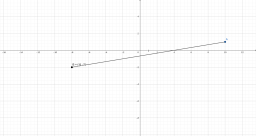# Evaluate expression

What is: the sum of negative four and one-half times n plus the quantity one-fourth plus one and three-fourths times n minus three-eighths?

x = -fr(27,4) + fr(7,4)n

### Step-by-step explanation:Did you find an error or inaccuracy? Feel free to write us. Thank you!

Tips for related online calculators
Need help calculating sum, simplifying, or multiplying fractions? Try our fraction calculator.
Need help with mixed numbers? Try our mixed-number calculator.